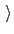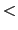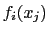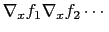Next: Biasing and analysis methods Up: Collective variable components (basis Previous: Linear and polynomial combinations   Contents   Index

## Colvars as scripted functions of components

When scripting is supported (default in VMD), a colvar may be defined as a custom function of its components, rather than a linear or polynomial combination. When implementing generic functions of Cartesian coordinates rather than functions of existing components, the cartesian component may be particularly useful.

An example of elaborate scripted colvar is given in example 10, in the form of path-based collective variables as defined by Branduardi et al. The required Tcl procedures are provided in the colvartools directory.

• scriptedFunctionCompute colvar as a scripted function of its componentsContext: colvar
Acceptable values: string
Description: If this option is specified, the colvar will be computed as a scripted function of the values of its components. To that effect, the user should define two Tcl procedures: calc_scriptedFunctionand calc_scriptedFunction_gradient, both accepting as many parameters as the colvar has components. Values of the components will be passed to those procedures in the order defined by their sorted name strings. Note that if all components are of the same type, their default names are sorted in the order in which they are defined, so that names need only be specified for combinations of components of different types. calc_scriptedFunctionshould return one value of typescriptedFunctionType, corresponding to the colvar value. calc_scriptedFunction_gradient should return a Tcl list containing the derivatives of the function with respect to each component. If both the function and some of the components are vectors, the gradient is really a Jacobian matrix that should be passed as a linear vector in row-major order, i.e. for a function:.

• scriptedFunctionTypeType of value returned by the scripted colvarContext: colvar
Acceptable values: string
Default value: scalar
Description: If a colvar is defined as a scripted function, its type is not constrained by the types of its components. With this flag, the user may specify whether the colvar is a scalar or one of the following vector types: vector3 (a 3D vector), unit_vector3 (a normalized 3D vector), or unit_quaternion (a normalized quaternion), or vector (a vector whose size is specified by scriptedFunctionVectorSize). Non-scalar values should be passed as space-separated lists, e.g. 1. 2. 3.''.

• scriptedFunctionVectorSizeDimension of the vector value of a scripted colvarContext: colvar
Acceptable values: positive integer
Description: This parameter is only valid when scriptedFunctionType is set to vector. It defines the vector length of the colvar value returned by the function.Next: Biasing and analysis methods Up: Collective variable components (basis Previous: Linear and polynomial combinations   Contents   Index
vmd@ks.uiuc.edu